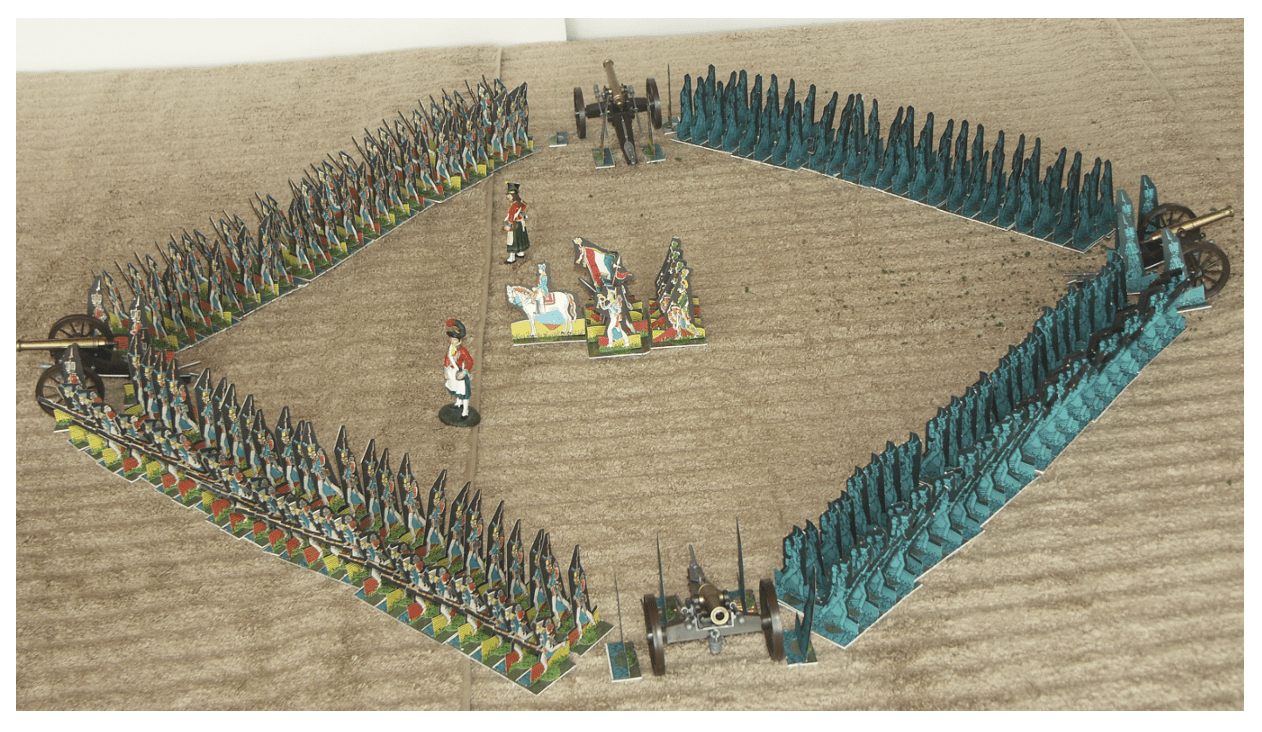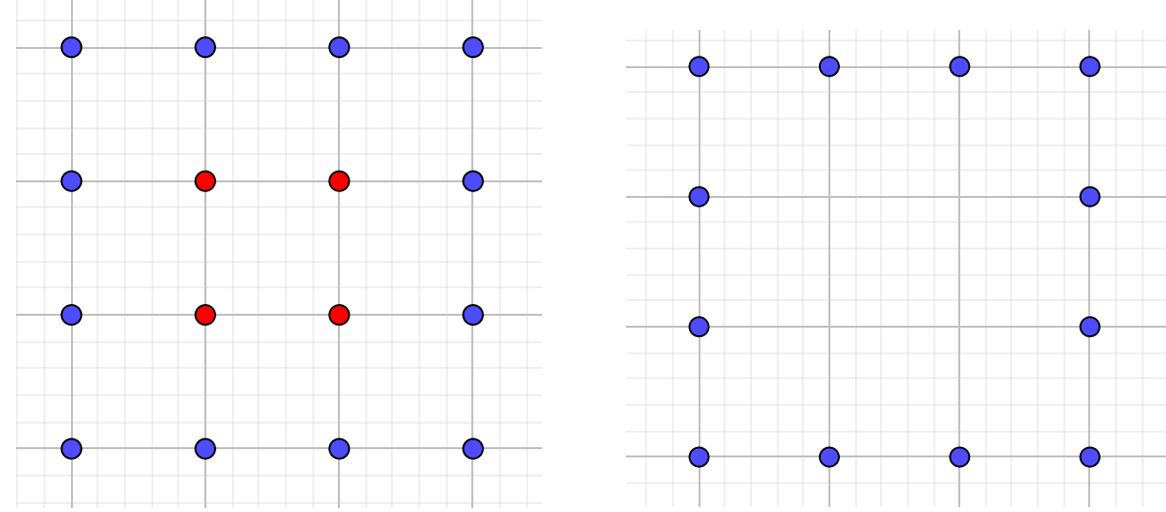# Starter of the Week 15: Hollow SquaresStarter of the Week 15: Hollow Squares

A hollow square was a battle formation used in the 1800s.  This consisted of a hollow square inside a larger square where the generals could safely command their troops.Here we can see that 12 men can be arranged in a hollow square formation (12 = 4 squared – 2 squared).

How many other numbers of men between 10 and 20 can be arranged in hollow squares?

Can you make a hypothesis about which numbers of men can’t be arranged in hollow squares?